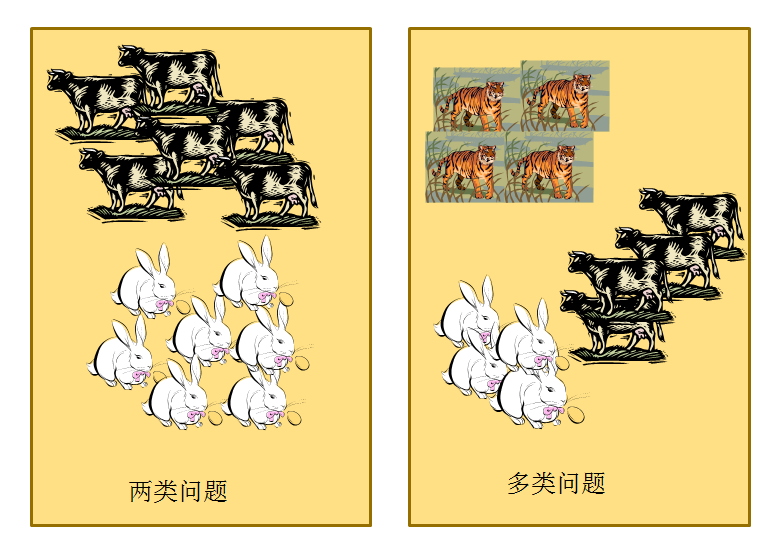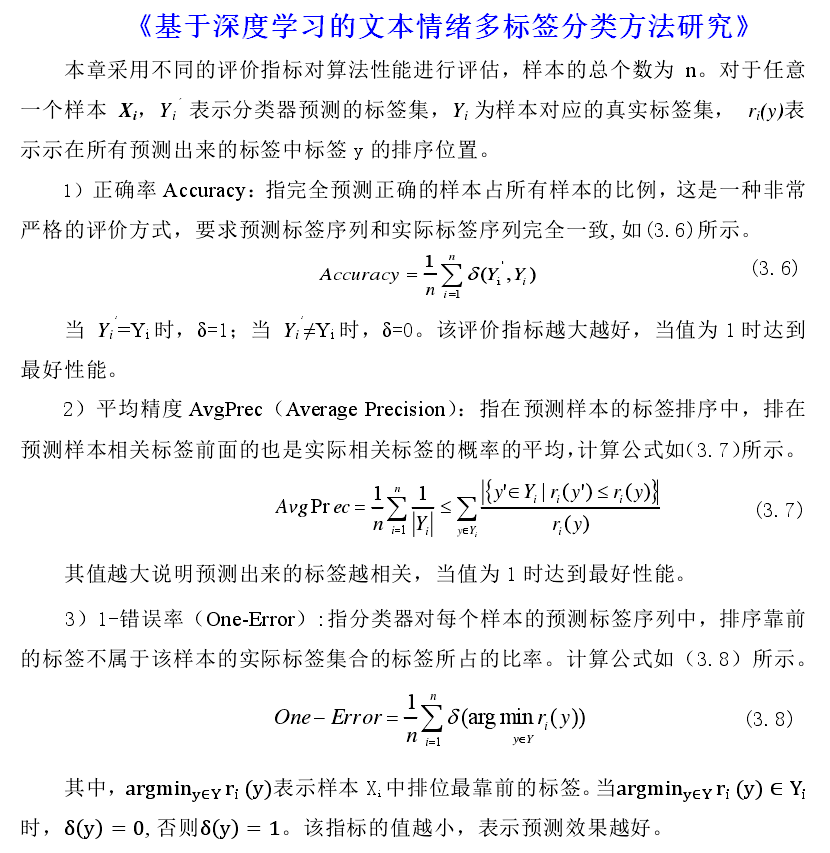• 多分类和多标签
千次阅读
2020-12-04 16:48:37

## 图像二分类，多分类和多标签分类的区别和注意事项

1. 二分类
分类任务是两个类别，每个样本属于两个类别中的一个，标签为0或者1，比如猫狗二分类
输出层：只有一个单元，采用sigmoid函数（将输出转换为0-1之间的概率分布）
损失函数：2分类交叉熵损失
标签：每个样本的标签是一个标量，0或者1

2. 多分类
分类任务有n个类别，每个样本属于n个类别中的一个，且每个样本有且只有一个标签，比如新闻题材分类：政治、经济、文化等
输出层：进行n分类时，设置输出层的单元数为n，采用softmax函数
损失函数：多分类交叉熵损失函数
标签：把标签转换为one-hot向量，每个样本的标签是一个n维向量，其所属类别位置是1，其余位置是0

3. 多标签分类
分类任务有n个类别，每个样本属于n个类别中的若干个，每个样本有若干个标签，比如一段新闻可以同时属于政治、经济等标签
输出层：采用sigmoid作为激活函数
损失函数：使用二进制交叉熵损失函数作为损失函数
标签：把标签转换为multi-hot向量，每个样本的标签是一个n维向量，其所属标签位置是1，其余位置是0
https://zhuanlan.zhihu.com/p/98322026神经网络 pytorch
更多相关内容
• 多标签分类(Multilabel classification): 给每个样本一系列的目标标签. 可以想象成一个数据点的各属性不是相互排斥的(一个水果既是苹果又是梨就是相互排斥的), 比如一个文档相关的话题. 一个文本可能被同时认为是...

# 1.基本概念

二分类：表示分类任务中有两个类别，比如我们想识别一幅图片是不是猫。也就是说，训练一个分类器，输入一幅图片，用特征向量x表示，输出是不是猫，用y=0或1表示。二类分类是假设每个样本都被设置了一个且仅有一个标签 0 或者 1。

多类分类(Multiclass classification): 表示分类任务中有多个类别, 比如对一堆水果图片分类, 它们可能是橘子、苹果、梨等. 多类分类是假设每个样本都被设置了一个且仅有一个标签: 一个水果可以是苹果或者梨, 但是同时不可能是两者。

多标签分类(Multilabel classification): 给每个样本一系列的目标标签. 可以想象成一个数据点的各属性不是相互排斥的(一个水果既是苹果又是梨就是相互排斥的), 比如一个文档相关的话题. 一个文本可能被同时认为是宗教、政治、金融或者教育相关话题。

# 2.分析两类问题是分类问题中最简单的一种。而很多多类问题可以被分解为多个两类问题进行求解。这幅图中有一栋房子、树、云、草，具有多个标签。多标签问题主要体现含有什么标签，而多分类问题主要体现区分出。
整个网络是fully connected全连接网络。

• 网络结构是输入层=你的特征的维度。

• 隐藏层是500*100，激励函数都是relu。隐藏层的节点数量和深度请根据自己的数量来自行调整，这里只是举例。

• 输出层是你的label的维度。使用sigmoid作为激励，使输出值介于0-1之间。

• 训练数据的label请用0和1的向量来表示。0代表这条数据没有这个位的label，1代表这条数据有这个位的label。假设3个label的向量[天空,人,大海]的向量值是[1,1,0]的编码的意思是这张图片有天空，有人，但是没有大海。

• 使用binary_crossentropy来进行损失函数的评价，从而在训练过程中不断降低交叉熵。实际变相的使1的label的节点的输出值更靠近1，0的label的节点的输出值更靠近0。

展开全文pytorch
• 分类：类别数目大于2个，类别之间是互斥的。比如是猫，就不能是狗、猪 多标签：类别之间不是...首先，说下多类分类和多标签分类的区别 多标签分类： 一个样本可以属于多个类别（或标签），不同类之间是有关联...

多分类：类别数目大于2个，类别之间是互斥的。比如是猫，就不能是狗、猪

多标签：类别之间不是互斥的。它有多个label，比如既有类别，又有位置信息等等

首先，说下多类分类和多标签分类的区别

多标签分类： 一个样本可以属于多个类别（或标签），不同类之间是有关联的，比如一个文本被被划分成“人物”和“体育人物”两个标签。很显然这两个标签不是互斥的，而是有关联的
多类分类：  一个样本属于且只属于多个分类中的一个，一个样本只能属于一个类，不同类之间是互斥的，比如一个文本只能被划分成“人物”，或者被划分成“文化”，而不能同时被划分成“人物”和“文化”，“文化”和“人物”这两个分类就是互斥的

那么，如何用softmax和sigmoid来做多类分类和多标签分类呢？

1、如何用softmax做多分类和多标签分类
现假设，神经网络模型最后的输出是这样一个向量logits=[1,2,3,4], 就是神经网络最终的全连接的输出。这里假设总共有4个分类
用softmax做多分类的方法：
tf.argmax(tf.softmax(logits))
首先用softmax将logits转换成一个概率分布，然后取概率值最大的作为样本的分类
这样看似乎，tf.argmax(logits)同样可以取得最大的值，也能得到正确的样本分类，这样的话softmax似乎作用不大
那么softmax的主要作用其实是在计算交叉熵上，首先样本集中y是一个one-hot向量，如果直接将模型输出logits和y来计算交叉熵，
因为logits=[1,2,3,4]，计算出来的交叉熵肯定很大，这种计算方式不对，而应该将logits转换成一个概率分布后再来计算，
就是用tf.softmax(logits)和y来计算交叉熵，当然我们也可以直接用tensorflow提供的方法sofmax_cross_entropy_with_logits来计算
这个方法传入的参数可以直接是logits，因为这个根据方法的名字可以看到，方法内部会将参数用softmax进行处理
现在我们取的概率分布中最大的作为最终的分类结果，这是多分类
我们也可以取概率的top几个，作为最终的多个标签，或者设置一个阈值，并取大于概率阈值的。这就用softmax实现了多标签分类

2、如何用sigmoid做多标签分类
sigmoid一般不用来做多类分类，而是用来做二分类的
它是将一个标量数字转换到[0,1]之间，如果大于一个概率阈值(一般是0.5)，则认为属于某个类别，否则不属于某个类别
那么如何用sigmoid来做多标签分类呢？其实就是针对logits中每个分类计算的结果分别作用一个sigmoid分类器，分别判定样本是否属于某个类别
同样假设，神经网络模型最后的输出是这样一个向量logits=[1,2,3,4], 就是神经网络最终的全连接的输出。这里假设总共有4个分类
tf.sigmoid(logits)
sigmoid应该会将logits中每个数字都变成[0,1]之间的概率值，假设结果为[0.01, 0.05, 0.4, 0.6],然后设置一个概率阈值，比如0.3，如果概率值大于0.3，则判定类别符合，那这里，样本会被判定为类别3和类别4都符合。

展开全文• 准确率-accuracy F1值 - fscore ...AUPRC - (很博客没有找到这个) 查准率- avgPrecision Intuitively, precision is the ability of the classifier not to label as positive a sample that多标签分类的分类评价指标分为两大类：

1. 基于标签上的度量：同多分类一样，在每一个标签上计算 Accuray、P、R、F……
2. 基于样本上的度量：又分为基于分类的度量、基于排序的度量
1. 基于分类的度量：Subset Accuracy、Hamming Loss、Accuracy exam、Precision exam、Recall exam、 F β F_{\beta} exam……
2. 基于排序的度量：one-error、Coverage、Ranking Loss、Average precision……多标签分类的评价指标可以划分为基于标签的评价指标基于样本的评价指标，区别在于：

• ① 基于标签的评价指标首先计算分类器在标签空间中每一个标签上的指标值，然后通过对全部标签上的评价值取宏观或微观平均得到最终指标值；
• ② 基于样本的评价指标首先评估分类器在每个样本上的表现，然后通过对测试集全部样本的指标值取平均来评估分类器在整个测试集上的表现。

# 一、基于标签上的度量（同多分类一样）## 1、Precision

Precision、 micro-P、macro-P、weighted-P

## 2、Recall

Recall、micro-Recall、macro-Recall、weighted-Recall

## 3、F1

F1、 micro-F1、macro-F1、weighted-F1

# 二、基于样本上的度量## 1、基于分类的度量

### 1.1 Hamming Loss（Hamming Distance）

h l o s s h_{loss} 可能是最直观也是最容易理解的一个loss，它直接统计了被误分类label的个数（不属于这个样本的标签被预测，或者属于这个样本的标签没有被预测）。 h l o s s = 0 h_{loss}=0 表示所有的每一个data的所有label都被分对了。
h l o s s = 1 p ∑ i = 1 p h ( x i ) Δ Y i ， 其 中 p = N × L h_{loss}= \frac{1}{p}∑_{i=1}^ph(x_{i})ΔY_{i}，其中 p=N\times L

图示：P：样本数 、Q：标签数 、 | ……|：错误样本的个数

例：HammingLoss用于考察样本在单个标记上的误分类情况，即相关标记未出现在预测的标记集合中或无关标记出现在预测的标记集合中(预测结果中，错误数/总数)。该指标取值越小则系统性能越优。

#computing hammingLoss
def HammingLossClass(preLabels,test_targets):
num_class,num_instance = np.mat(test_targets).shape
temp = sum((preLabels != test_targets))
miss_pairs = sum(temp)
hammingLoss = miss_pairs/(num_class*num_instance)
return hammingLoss


## 2、基于排序的度量### 2.1 查准率- Average precision

Intuitively, precision is the ability of the classifier not to label as positive a sample that is negative, and recall is the ability of the classifier to find all the positive samples（查准率是分类器不把阴性样本标记为阳性的能力，而召回率是分类器找到所有阳性样本的能力。）2者都是越高越好.
个人关于查准率和召回率简记（都是基于多分类为背景），最好结合一个混淆矩阵读下面例子：
查准率：字面意思，希望查的越准越好，识别模型查的准不准。举个极端例子，模型预测该类99个样本为真（99/100， 100个样本下），但实际只有5个样本。此时prec= 5/99, 查准率就非常小了。说明模型查的不准，因为它把很多阴性样本标记为阳性样本。
召回率：字面意思，召回，希望召回产品越来越少（比如说，研制了A产品，公司觉得是好产品（你的模型预测为真），但是，消费者一使用，发现很多是坏的（实际是假），此时需要重新召回产品，重新修理，然后在给消费者。）。 召回率越高，说明公司真正召回产品的概率越低，即1-recall。

### 2.4 秩损失 -ranking_loss

# -*- coding: utf-8 -*-
"""
Created on Sun Sep  6 20:38:38 2020
"""
#from sklearn import datasets
import sklearn
#import torch
import numpy as np
from scipy.sparse import csr_matrix
from scipy.sparse.csgraph import laplacian
from scipy.sparse.linalg import eigs
from sklearn.metrics import accuracy_score
from sklearn.metrics import f1_score
from sklearn.metrics import hamming_loss
from sklearn.metrics import roc_auc_score
from sklearn.metrics import precision_score, recall_score,auc

# np.set_printoptions(threshold='nan')
class Metric(object):
def __init__(self,output,label):
self.output = output   #prediction label matric
self.label = label    #true  label matric

def accuracy_subset(self,threash=0.5):
y_pred =self.output
y_true = self.label
y_pred=np.where(y_pred>threash,1,0)
accuracy=accuracy_score(y_true,y_pred)
return accuracy

def accuracy_mean(self,threash=0.5):
y_pred =self.output
y_true = self.label
y_pred=np.where(y_pred>threash,1,0)
accuracy=np.mean(np.equal(y_true,y_pred))
return accuracy

def accuracy_multiclass(self):
y_pred =self.output
y_true = self.label
accuracy=accuracy_score(np.argmax(y_pred,1),np.argmax(y_true,1))
return accuracy

def micfscore(self,threash=0.5,type='micro'):
y_pred =self.output
y_true = self.label
y_pred=np.where(y_pred>threash,1,0)
return f1_score(y_pred,y_true,average=type)
def macfscore(self,threash=0.5,type='macro'):
y_pred =self.output
y_true = self.label
y_pred=np.where(y_pred>threash,1,0)
return f1_score(y_pred,y_true,average=type)

def hamming_distance(self,threash=0.5):
y_pred =self.output
y_true = self.label
y_pred=np.where(y_pred>threash,1,0)
return hamming_loss(y_true,y_pred)

def fscore_class(self,type='micro'):
y_pred =self.output
y_true = self.label
return f1_score(np.argmax(y_pred,1),np.argmax(y_true,1),average=type)

def auROC(self):
y_pred =self.output
y_true = self.label
row,col = label.shape
temp = []
ROC = 0
for i in range(col):
sigle_ROC = roc_auc_score(y_true[:,i], y_pred[:,i], average='macro', sample_weight=None)
#print("%d th AUROC: %f"%(i,ROC))
temp.append(sigle_ROC)

ROC += sigle_ROC
return ROC/(col)

def MacroAUC(self):
y_pred =self.output #num_instance*num_label
y_true = self.label #num_instance*num_label
num_instance,num_class =   y_pred.shape
count = np.zeros((num_class,1))   # store the number of postive instance'score>negative instance'score
num_P_instance =  np.zeros((num_class,1)) #number of positive instance for every label
num_N_instance =  np.zeros((num_class,1))
AUC = np.zeros((num_class,1))  # for each label
count_valid_label = 0
for  i in range(num_class): #第i类
num_P_instance[i,0] = sum(y_true[:,i] == 1) #label,,test_target
num_N_instance[i,0] = num_instance - num_P_instance[i,0]
# exclude the label on which all instances are positive or negative,
# leading to num_P_instance(i,1) or num_N_instance(i,1) is zero
if num_P_instance[i,0] == 0 or num_N_instance[i,0] == 0:
AUC[i,0] = 0
count_valid_label = count_valid_label + 1
else:

temp_P_Outputs = np.zeros((int(num_P_instance[i,0]), num_class))
temp_N_Outputs = np.zeros((int(num_N_instance[i,0]), num_class))
#
temp_P_Outputs[:,i] = y_pred[y_true[:,i]==1,i]
temp_N_Outputs[:,i] = y_pred[y_true[:,i]==0,i]
for m in range(int(num_P_instance[i,0])):
for n in range(int(num_N_instance[i,0])):
if(temp_P_Outputs[m,i] > temp_N_Outputs[n,i] ):
count[i,0] = count[i,0] + 1
elif(temp_P_Outputs[m,i] == temp_N_Outputs[n,i]):
count[i,0] = count[i,0] + 0.5

AUC[i,0] = count[i,0]/(num_P_instance[i,0]*num_N_instance[i,0])
macroAUC1 = sum(AUC)/(num_class-count_valid_label)
return  float(macroAUC1),AUC

def avgPrecision(self):
y_pred =self.output
y_true = self.label
num_instance,num_class =  y_pred.shape
precision_value = 0
precisions = []
for i in range(num_instance):
p = precision_score(y_true[i,:], y_pred[i,:])
precisions.append(p)
precision_value += p
#print(precision_value)
pre_list = np.array([1.0] + precisions + [0.0] )#for get AUPRC
#print(pre_list)
return float(precision_value/num_instance), pre_list

def avgRecall(self):
y_pred =self.output
y_true = self.label
num_instance,num_class =  y_pred.shape
recall_value = 0
recalls  = []
for i in range(num_instance):
p = recall_score(y_true[i,:], y_pred[i,:])
recalls.append(p)
recall_value += p
rec_list = np.array([0.0] + recalls + [1.0]) #for get AUPRC
sorting_indices = np.argsort(rec_list)
#print(rec_list)
return float(recall_value/num_instance),rec_list,sorting_indices

def getAUPRC(self):
avgPrecision,precisions = self.avgPrecision()
avfRecall,recalls, sorting_indices  = self.avgRecall()
#x is either increasing or decreasing
#such as recalls[sorting_indices]
auprc = auc(recalls[sorting_indices], precisions[sorting_indices])
return auprc

def cal_single_label_micro_auc(self,x, y):
idx = np.argsort(x)  # 升序排列
y = y[idx]
m = 0
n = 0
auc = 0
for i in range(x.shape):
if y[i] == 1:
m += 1
auc += n
if y[i] == 0:
n += 1
auc /= (m * n)
return auc

def get_micro_auc(self):
"""
:param x: the predicted outputs of the classifier, the output of the ith instance for the jth class is stored in x(i,j)
:param y: the actual labels of the instances, if the ith instance belong to the jth class, y(i,j)=1, otherwise y(i,j)=0
:return: the micro auc
"""
x =self.output
y = self.label
n, d = x.shape
if x.shape != y.shape:
print("num of  instances for output and ground truth is different!!")
if x.shape != y.shape:
print("dim of  output and ground truth is different!!")
x = x.reshape(n * d)
y = y.reshape(n * d)
auc = self.cal_single_label_micro_auc(x, y)
return auc

def cal_single_instance_coverage(self,x, y):
idx = np.argsort(x)  # 升序排列
y = y[idx]
loc = x.shape
for i in range(x.shape):
if y[i] == 1:
loc -= i
break
return loc

def get_coverage(self):
"""
:param x: the predicted outputs of the classifier, the output of the ith instance for the jth class is stored in x(i,j)
:param y: the actual labels of the test instances, if the ith instance belong to the jth class, y(i,j)=1, otherwise y(i,j)=0
:return: the coverage
"""
x =self.output
y = self.label
n, d = x.shape
if x.shape != y.shape:
print("num of  instances for output and ground truth is different!!")
if x.shape != y.shape:
print("dim of  output and ground truth is different!!")
cover = 0
for i in range(n):
cover += self.cal_single_instance_coverage(x[i], y[i])
cover = cover / n - 1
return cover

def cal_single_instance_ranking_loss(self,x, y):
idx = np.argsort(x)  # 升序排列
y = y[idx]
m = 0
n = 0
rl = 0
for i in range(x.shape):
if y[i] == 1:
m += 1
if y[i] == 0:
rl += m
n += 1
rl /= (m * n)
return rl

def get_ranking_loss(self):
"""
:param x: the predicted outputs of the classifier, the output of the ith instance for the jth class is stored in x(i,j)
:param y: the actual labels of the test instances, if the ith instance belong to the jth class, y(i,j)=1, otherwise x(i,j)=0
:return: the ranking loss
"""
x =self.output
y = self.label
n, d = x.shape
if x.shape != y.shape:
print("num of  instances for output and ground truth is different!!")
if x.shape != y.shape:
print("dim of  output and ground truth is different!!")
m = 0
rank_loss = 0
for i in range(n):
s = np.sum(y[i])
if s in range(1, d):
rank_loss += self.cal_single_instance_ranking_loss(x[i], y[i])
m += 1
rank_loss /= m
return rank_loss

if __name__ == '__main__':
# 6行5列，6个样本，5个类别标记
output = np.array([[1,0,0,0,1],
[1,1,0,1,0],
[0,1,0,0,1],
[1,0,1,0,1],
[1,0,1,1,1],
[1,1,0,0,1]
])

label = np.array([[1,0,1,0,1],
[1,1,0,1,0],
[0,1,0,0,1],
[0,1,0,0,1],
[0,0,1,0,1],
[1,1,0,0,1]
])
myMetic = Metric(output,label)
#Macrof1 = myMetic.fscore_class()
ham = myMetic.hamming_distance()
Microf1 = myMetic.micfscore()
Macrof1 = myMetic.macfscore()
AUROC = myMetic.auROC()
MacroAUROC1,AUC_list = myMetic.MacroAUC()
avgPrecision,precisions = myMetic.avgPrecision()
avfRecall,recalls,sorting_indices  = myMetic.avgRecall()
auprc  = myMetic.getAUPRC()
#!!!!!!!!!!!!!!!!!!!!!!!!!!!!!!!!!!!!!!!!!!!!!!!!!!!!!!!!!!
micro_auc = myMetic.get_micro_auc()
coverage = myMetic.get_coverage()
ranking_loss = myMetic.get_ranking_loss()
#    #print(Macrof1)
print("ham:",ham)
print("Microf1:",Microf1)
print("Macrof1:",Macrof1)
print("AUROC: ",(AUROC))
print("MacroAUC: ",(MacroAUROC1))
#    #print(": ",(AUC_list))
print("avgPrecision: ",avgPrecision)
print("avfRecall: ",avfRecall)
print("AUPRC: ",auprc)
print("get_micro_auc _from_KDD2018M3DS:",micro_auc)
print("get_coverage _from_KDD2018M3DS:",coverage)
print("get_ranking_loss _from_KDD2018M3DS:",ranking_loss)
#    #iris = datasets.load_iris()



打印结果：

ham: 0.2
Microf1: 0.8125
Macrof1: 0.7547619047619046
AUROC:  0.8133333333333332
MacroAUC:  0.8133333333333332
avgPrecision:  0.8055555555555557
avfRecall:  0.861111111111111
AUPRC:  0.7777777777777778
get_micro_auc _from_KDD2018M3DS: 0.8
get_coverage _from_KDD2018M3DS: 2.3333333333333335
get_ranking_loss _from_KDD2018M3DS: 0.13888888888888887

Process finished with exit code 0


展开全文分类 人工智能 评价指标
• 2、Multi-Label：多标签分类 文本可能同时涉及任何宗教，政治，金融或教育，也可能不属于任何一种。 电影可以根据其摘要内容分为动作，喜剧浪漫类型。有可能电影属于romcoms [浪漫与喜剧]等多种类型。 例子：
•   本文是一篇中文综述，主要介绍了层次多标签分类的一些算法。 ·参考文献：    赵海燕，曹杰，陈庆奎，曹健．层次多标签文本分类方法[J/OL]．小型微型计 算机系统. ...层级文本分类
• 分类一般分为三种情况：二分类、多分类和多标签分类。多标签分类比较直观的理解是，一个样本可以同时拥有几个类别标签，比如一首歌的标签可以是流行、轻快，一部电影的标签可以是动作、喜剧、搞笑，一本书的标签可以...分类
• 标签多分类问题，指一个样本（一个图片或者一个候选框）有一个标签，但总共的类别数是类的。 比如常见的OCR问题，目标检测中针对每个候选框的分类多分类问题。 在深度学习中，softmax是最常用的解决方案。 ...机器学习 计算机视觉 神经网络
• 任务学习可以运用到许多的场景。 首先，任务学习可以学到个任务的共享表示，这个共享表示具有较强的抽象能力，能够适应个不同但相关的目标，通常...相关任务任务学习二分类多分类多标签分类相关关系 相关语音识别 神经网络 多分类 多任务学习
•   本文在BERT模型上，提出了利用任务架构来解决层级多标签文本分类问题。 ·参考文献：    MSML-BERT模型的层级多标签文本分类方法研究  摘要   在摘要中，作者主要给出了本篇论文的创新点：   1...
• 标签分类算法，单标签多分类算法，多标签多分类算法及多标签多分类在Scikit-learn中的实现方式。
• 我们先用sklearn生成一个多标签分类数据集。 from sklearn.datasets import make_multilabel_classification X, y = make_multilabel_classification(n_samples=1000, n_features=10, n_classes=pytorch
• 多标签分类在数据科学中是一个比较令人头疼的问题。在这篇文章中，我将给你一个直观的解释，说明什么是多标签分类，以及如何解决这个问题。1.多标签分类是什么?让我们来看看下面的图片。如果我问你这幅图中有一栋...
• 文本分类一般可以分为二分类多分类多标签分类三种情况,二分类是指将一组文本分成两个类(0或1),比较常见的应用如垃圾邮件分类、电商网站的用户评价数据的正负面分类等,多分类是指将文本分成若干个类中的某一个类,...分类算法评估
• 详解sigmoid与softmax， 多分类多标签分类激活函数介绍sigmoid激活函数sigmoid激活函数的性质sigmoid激活函数的使用 激活函数介绍 对于熟悉机器学习或神经网络的读者来说，sigmoid与softmax两个激活函数并不陌生，...softmax 多分类
• 将路透社新闻划分为 46 个互斥的主题...如果每个数据点可以划分到个类别（主题），那它就是一个多标签多分类（multilabel, multiclass classification）问题。 1 单标签多分类问题模型的训练 单标签多分类问题有以keras 单标签多分类
• 前面在我的博客中所讲的内容是单标签多分类，例如一个手写数字图片，分出10类，得到这个图片表示的1个字符信息，而验证码一般是个字符，每一个字符均有个类别，因此验证码识别的问题就属于多标签多分类问题，在...keras 多标签多分类
• ## CNN图像多标签分类

千次阅读 2019-10-14 15:00:27
正像是一个神经网络没法预测出一个它未曾训练过的类，你的神经网络没法预测出它未曾见过的标签组合。这个特性是由于神经网络内部神经元的激活函数。 如果你的神经网络同时经过：（1）黑色裤子（2）红色衬衫的...
• ## 多标签分类

万次阅读 2019-06-29 18:24:55
如文本分类、图像类别标注、语义场景分类等一些实际应用中，常常会出现一个示例同时属于多个类别（比如:一张电影海报图片可能会同时有科幻、动作、喜剧等多个标签），下图展示了单标签分类和多标签分类之间的区别。...
• 首先，说下多类分类和多标签分类的区别 多标签分类： 一个样本可以属于多个类别（或标签），不同类之间是有关联的，比如一个文本被被划分成“人物”和“体育人物”两个标签。很显然这两个标签不是互斥的，而是有...
• 【人工智能项目】深度学习实现图像多标签分类 本次实现一个图像多标签分类的任务，接下来还会分享我研究生阶段做过的其它任务，走起瓷！！！ 任务介绍 训练一个多标签分类模型，使得该模型能自动根据输入的任意...分类 人工智能
• 相信很小伙伴最开始都是从分类任务入手深度学习这个领域的吧，这个就类似学习代码的第一课，“Hello world”一样。深度学习中，除了模型设计之外，最重要的想必就是选取合适的损失函数了。不过一般实验中，损失...分类 pytorch 数据挖掘
• 标签多分类算法 Softmax算法 One-Versus-One（ovo）：一对一 One-Versus-All / One-Versus-the-Rest（ova/ovr）： 一对 ovoovr的区别 Error Correcting Output codes（纠错码机制）： 多标签多...
• 多标签文本分类 Bert简介 两个任务 Bert是按照两个任务进行预训练的，分别是遮蔽语言任务(Masked Language Model)句子预测任务(NextSentence Prediction)。 遮蔽语言任务（Masked Language Model） 对输入的语句中...bert 分类 自然语言处理 pytorch
• 3. 多标签分类问题 参考文章 1. 基本概念 2分类 分类任务有两个类别，每个样本属于两个类别中的一个，标签0/1.比如：训练一个图像分类器，判断一张输入图片是否是猫。 多分类 分类任务有n个类别，每个样本...多分类
• 解决多标签多分类中损失函数选择及样本不均衡问题的2个帖子 https://cloud.tencent.com/developer/ask/226097 https://discuss.pytorch.org/t/multi-label-multi-class-class-imbalance/37573 主要弄明白 nn....pytorch 多标签多分类
• 多标签分类器  多标签分类任务与多分类任务有所不同，多分类任务是将一个实例分到某个类别中，多标签分类任务是将某个实例分到个类别中。多标签分类任务有有两大特点： 类标数量不确定，有些样本可能只有一个类标...机器学习 分类
• 多标签分类 评价指标Metrics play quite an important role in the field of Machine Learning or Deep Learning. We start the problems with metric selection as to know the baseline score of a particular ...机器学习 python 人工智能 大数据
• 我们将使用Keras训练多标签分类器来预测服装的颜色类型 我们的数据集包含 六个类别 的 2,167 个图像，包括： 黑色牛仔裤（344张） 蓝色连衣裙（386张） 蓝色牛仔裤（356张） 蓝色衬衫（369张） ...Keras
• 目的： 训练一个分类器来将物品分到不同的类别中，比如一件衣服：可以安照服饰...首先讨论多标签分类数据集（以及如何快速构建自己的数据集）。 之后简要讨论SmallerVGGNet，我们将实现的Keras神经网络架构，并...Keras...